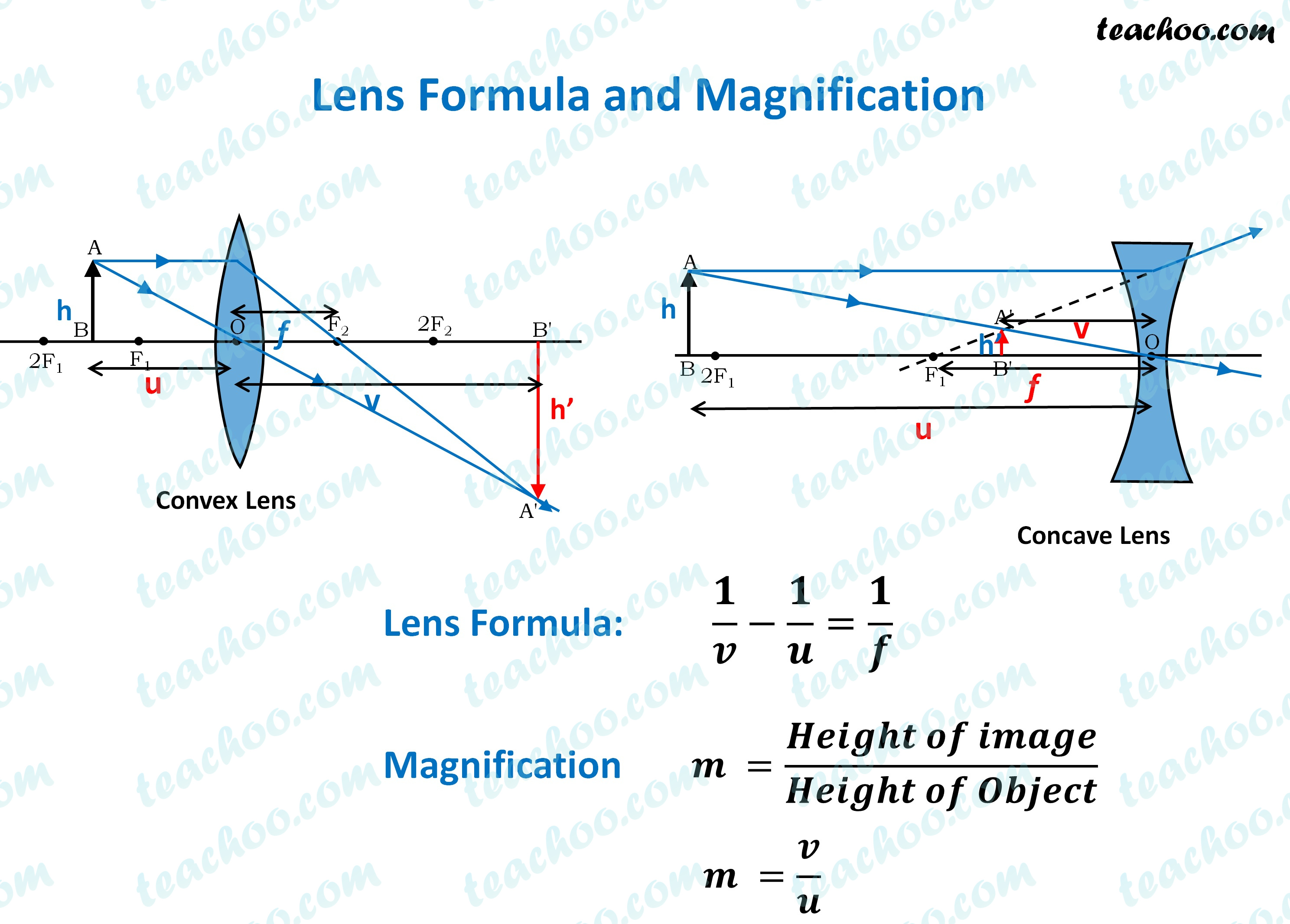Concepts

Class 10
Chapter 10 Class 10 - Light - Reflection and Refraction

## What is Object Distance

Distance of Object from Optical Centre of the lens is called Object Distance

It is denoted by u

## What is Image Distance

Distance of Image from Optical Centre of the lens is called Object Distance

It is denoted by v.

## What is Focal length

Distance of Principal Focus from the optical centre of the lens is Called Focal Length

It is denoted by f

## What is lens Formula

This formula denotes relationship between

• Object Distance (u)
• Image Distance (v)
• Focal length (f)

##googletag.cmd.push(function() { googletag.display('div-gpt-ad-1669298377854-0'); });

Note : - We follow the sign convention for lenses
In the image, the red text is negative, and blue text is positive

Thus,

• If Virtual image is formed,v is negative
• If Real Image is formed, v is positive
• Object Distance (u) is always negative as it is on left side of the lens
• Focal Length of Convex Lens is Positive
• Focal Length of Concave Lens is Negative

In addition to the mirror formula,

we have the magnification formula

## What is the magnification formula?

It is Ratio of Height of Image to the Height of Object

Hence it gives extent image is magnified compared to image of object

Suppose Height of object is h

Height of Image is h'

Magnification is m

So

Magnification = Height of Image/ Height of Object

m = h'/h

We also have another formula for magnification in lenses

Magnification = v/u

where

v is image distance

u is object distance

Note: -  If magnification (m) is positive, It means image formed is virtual and erect
If magnification (m) is negative, It means image formed is real and inverted

## Questions

Example 10.3 - A concave lens has focal length of 15 cm. At what distance should the object from the lens be placed so that it forms an image at 10 cm from the lens? Also, find the magnification produced by the lens.

Example 10.4 - A 2.0 cm tall object is placed perpendicular to the principal axis of a convex lens of focal length 10 cm. The distance of the object from the lens is 15 cm. Find the nature, position and size of the image. Also find its magnification.

NCERT Question 9 - One-half of a convex lens is covered with a black paper. Will this lens produce a complete image of the object? Verify your answer experimentally. Explain your observations.

NCERT Question 10 - An object 5 cm in length is held 25 cm away from a converging lens of focal length 10 cm. Draw the ray diagram and find the position, size and the nature of the image formed

NCERT Question 11 - A concave lens of focal length 15 cm forms an image 10 cm from the lens. How far is the object placed from the lens? Draw the ray diagram.

Learn in your speed, with individual attention - Teachoo Maths 1-on-1 Class